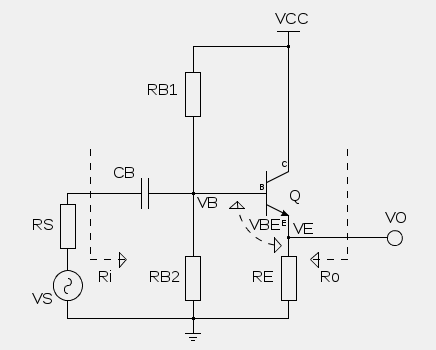OVERVIEW This is a simple design tool for calculating bias resistor values, small-signal gain and input/output resistances of an emitter follower BJT amplifier. The emitter follower is typically used as a buffer, which provides high input impedance and low output impedance. Just fill the input fields below in given order from top to bottom. The ordering of the fields serves as a step-by-step guide for the design process. The base bias resistor values are calculated automatically (using 5% tolerance resistances), but custom values can be given and those will not be overwritten. After iterating any resistance value, always press 'Calculate results' button to update the results. The small-signal values are evaluated in the mid-band, CB short-circuited. The small-signal gain is evaluated as VO/VS, and the input/output resistances are evaluated as shown in the pic.Select the operating voltage VCC. Typically 9 volts is the way to go. VCC: volts RESULTS DC Bias voltages: VC : volts VE : volts VB : volts AC Voltage Gain factor: Av : const    dB AC Input resistance: Ri : ohms AC Output resistance: Ro : ohms Select the BJT you want to use, from its datasheet lookup the current gain factor and place it here as a parameter. hFE: const For convenience, you can change the base-emitter voltage, which is set to 0.65 V by default. Typical VBE is 0.55 - 0.75 V. VBE: volts Choose the emitter bias voltage to aim for. Typically the collector bias voltage is half of the operating voltage VCC. VE: volts Choose the emitter resistor RE for better bias stability and input impedance. The value of RE affects the base bias resistor values and input impedance. RE: ohms A multiplier for base resistor values, which are obtained in the next step. Too much resistance at the base affects the stability of biasing. Use values between 4 - 16. Bx: const Use 'Calculate values' button to evaluate exact values for base resistors. Then use the arrow buttons to round up or down to nearest standard value, or leave as is. RB1:RB2: ohms ohms Also, the internal resistance of the signal source should be given. For ideal source this value is small, but for guitar output this is a few kilohms. RS : ohms